Elementary Algebra

### Learning Objectives

By the end of this section, you will be able to:

• Recognize the graph of a quadratic equation in two variables
• Find the axis of symmetry and vertex of a parabola
• Find the intercepts of a parabola
• Graph quadratic equations in two variables
• Solve maximum and minimum applications

### Be Prepared 10.5

Before you get started, take this readiness quiz.

1. Graph the equation $y=3x−5y=3x−5$ by plotting points.
If you missed this problem, review Example 4.11.
2. Evaluate $2x2+4x−12x2+4x−1$ when $x=−3x=−3$.
If you missed this problem, review Example 1.57.
3. Evaluate $−b2a−b2a$ when $a=13a=13$ and $b=56b=56$.
If you missed this problem, review Example 1.89.

### Recognize the Graph of a Quadratic Equation in Two Variables

We have graphed equations of the form $Ax+By=CAx+By=C$. We called equations like this linear equations because their graphs are straight lines.

Now, we will graph equations of the form $y=ax2+bx+cy=ax2+bx+c$. We call this kind of equation a quadratic equation in two variables.

### Quadratic Equation in Two Variables

A quadratic equation in two variables, where $a,b,andca,b,andc$ are real numbers and $a≠0a≠0$, is an equation of the form

$y=ax2+bx+cy=ax2+bx+c$

Just like we started graphing linear equations by plotting points, we will do the same for quadratic equations.

Let’s look first at graphing the quadratic equation $y=x2y=x2$. We will choose integer values of $xx$ between $−2−2$ and 2 and find their $yy$ values. See Table 10.1.

 $y=x2y=x2$ $xx$ $yy$ 0 0 1 1 $−1−1$ 1 2 4 $−2−2$ 4
Table 10.1

Notice when we let $x=1x=1$ and $x=−1x=−1$, we got the same value for $yy$.

$y=x2y=x2y=12y=(−1)2y=1y=1y=x2y=x2y=12y=(−1)2y=1y=1$

The same thing happened when we let $x=2x=2$ and $x=−2x=−2$.

Now, we will plot the points to show the graph of $y=x2y=x2$. See Figure 10.2.

Figure 10.2

The graph is not a line. This figure is called a parabola. Every quadratic equation has a graph that looks like this.

In Example 10.43 you will practice graphing a parabola by plotting a few points.

### Example 10.43

Graph $y=x2−1y=x2−1$.

### Try It 10.85

Graph $y=−x2y=−x2$.

### Try It 10.86

Graph $y=x2+1y=x2+1$.

How do the equations $y=x2y=x2$ and $y=x2−1y=x2−1$ differ? What is the difference between their graphs? How are their graphs the same?

All parabolas of the form $y=ax2+bx+cy=ax2+bx+c$ open upwards or downwards. See Figure 10.3.

Figure 10.3

Notice that the only difference in the two equations is the negative sign before the $x2x2$ in the equation of the second graph in Figure 10.3. When the $x2x2$ term is positive, the parabola opens upward, and when the $x2x2$ term is negative, the parabola opens downward.

### Parabola Orientation

For the quadratic equation $y=ax2+bx+cy=ax2+bx+c$, if: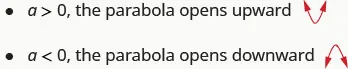### Example 10.44

Determine whether each parabola opens upward or downward:

$y=−3x2+2x−4y=−3x2+2x−4$ $y=6x2+7x−9y=6x2+7x−9$

### Try It 10.87

Determine whether each parabola opens upward or downward:

$y=2x2+5x−2y=2x2+5x−2$ $y=−3x2−4x+7y=−3x2−4x+7$

### Try It 10.88

Determine whether each parabola opens upward or downward:

$y=−2x2−2x−3y=−2x2−2x−3$ $y=5x2−2x−1y=5x2−2x−1$

### Find the Axis of Symmetry and Vertex of a Parabola

Look again at Figure 10.3. Do you see that we could fold each parabola in half and that one side would lie on top of the other? The ‘fold line’ is a line of symmetry. We call it the axis of symmetry of the parabola.

We show the same two graphs again with the axis of symmetry in red. See Figure 10.4.

Figure 10.4

The equation of the axis of symmetry can be derived by using the Quadratic Formula. We will omit the derivation here and proceed directly to using the result. The equation of the axis of symmetry of the graph of $y=ax2+bx+cy=ax2+bx+c$ is $x=−b2a.x=−b2a.$

So, to find the equation of symmetry of each of the parabolas we graphed above, we will substitute into the formula $x=−b2ax=−b2a$.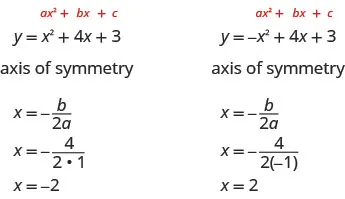Look back at Figure 10.4. Are these the equations of the dashed red lines?

The point on the parabola that is on the axis of symmetry is the lowest or highest point on the parabola, depending on whether the parabola opens upwards or downwards. This point is called the vertex of the parabola.

We can easily find the coordinates of the vertex, because we know it is on the axis of symmetry. This means its x-coordinate is $−b2a−b2a$. To find the y-coordinate of the vertex, we substitute the value of the x-coordinate into the quadratic equation.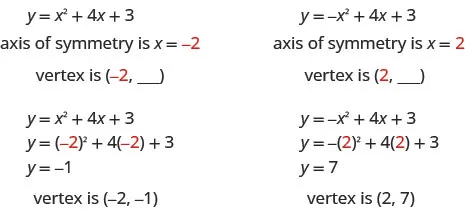### Axis of Symmetry and Vertex of a Parabola

For a parabola with equation $y=ax2+bx+cy=ax2+bx+c$:

• The axis of symmetry of a parabola is the line $x=−b2ax=−b2a$.
• The vertex is on the axis of symmetry, so its x-coordinate is $−b2a−b2a$.

To find the y-coordinate of the vertex, we substitute $x=−b2ax=−b2a$ into the quadratic equation.

### Example 10.45

For the parabola $y=3x2−6x+2y=3x2−6x+2$ find: the axis of symmetry and the vertex.

### Try It 10.89

For the parabola $y=2x2−8x+1y=2x2−8x+1$ find: the axis of symmetry and the vertex.

### Try It 10.90

For the parabola $y=2x2−4x−3y=2x2−4x−3$ find: the axis of symmetry and the vertex.

### Find the Intercepts of a Parabola

When we graphed linear equations, we often used the x- and y-intercepts to help us graph the lines. Finding the coordinates of the intercepts will help us to graph parabolas, too.

Remember, at the y-intercept the value of $xx$ is zero. So, to find the y-intercept, we substitute $x=0x=0$ into the equation.

Let’s find the y-intercepts of the two parabolas shown in the figure below.

Figure 10.5

At an x-intercept, the value of $yy$ is zero. To find an x-intercept, we substitute $y=0y=0$ into the equation. In other words, we will need to solve the equation $0=ax2+bx+c0=ax2+bx+c$ for $xx$.

$y=ax2+bx+c0=ax2+bx+cy=ax2+bx+c0=ax2+bx+c$

But solving quadratic equations like this is exactly what we have done earlier in this chapter.

We can now find the x-intercepts of the two parabolas shown in Figure 10.5.

First, we will find the x-intercepts of a parabola with equation $y=x2+4x+3y=x2+4x+3$.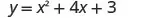Let $y=0y=0$.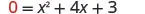Factor.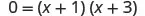Use the zero product property.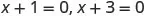Solve.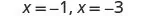The x intercepts are $(−1,0)(−1,0)$ and $(−3,0).(−3,0).$

Now, we will find the x-intercepts of the parabola with equation $y=−x2+4x+3y=−x2+4x+3$.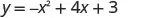Let $y=0y=0$.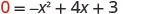This quadratic does not factor, so we use the Quadratic Formula.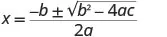$a=−1a=−1$, $b=4b=4$, $c=3c=3$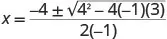Simplify.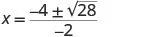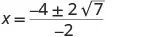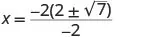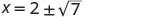The x intercepts are $(2+7,0)(2+7,0)$ and $(2−7,0)(2−7,0)$.

We will use the decimal approximations of the x-intercepts, so that we can locate these points on the graph.

$(2+7,0)≈(4.6,0)(2−7,0)≈(−0.6,0)(2+7,0)≈(4.6,0)(2−7,0)≈(−0.6,0)$

Do these results agree with our graphs? See Figure 10.6.

Figure 10.6

### How To

#### Find the intercepts of a parabola.

To find the intercepts of a parabola with equation $y=ax2+bx+cy=ax2+bx+c$:

$y-interceptx-interceptsLetx=0and solve fory.Lety=0and solve forx.y-interceptx-interceptsLetx=0and solve fory.Lety=0and solve forx.$

### Example 10.46

Find the intercepts of the parabola $y=x2−2x−8y=x2−2x−8$.

### Try It 10.91

Find the intercepts of the parabola $y=x2+2x−8.y=x2+2x−8.$

### Try It 10.92

Find the intercepts of the parabola $y=x2−4x−12.y=x2−4x−12.$

In this chapter, we have been solving quadratic equations of the form $ax2+bx+c=0ax2+bx+c=0$. We solved for $xx$ and the results were the solutions to the equation.

We are now looking at quadratic equations in two variables of the form $y=ax2+bx+cy=ax2+bx+c$. The graphs of these equations are parabolas. The x-intercepts of the parabolas occur where $y=0y=0$.

For example:

$Quadratic equationQuadratic equation in two variablesy=x2−2x−15x2−2x−15=0(x−5)(x+3)=0lety=00=x2−2x−150=(x−5)(x+3)x−5=0x+3=0x=5x=−3x−5=0x+3=0x=5x=−3(5,0)and(−3,0)x-interceptsQuadratic equationQuadratic equation in two variablesy=x2−2x−15x2−2x−15=0(x−5)(x+3)=0lety=00=x2−2x−150=(x−5)(x+3)x−5=0x+3=0x=5x=−3x−5=0x+3=0x=5x=−3(5,0)and(−3,0)x-intercepts$

The solutions of the quadratic equation are the $xx$ values of the x-intercepts.

Earlier, we saw that quadratic equations have 2, 1, or 0 solutions. The graphs below show examples of parabolas for these three cases. Since the solutions of the equations give the x-intercepts of the graphs, the number of x-intercepts is the same as the number of solutions.

Previously, we used the discriminant to determine the number of solutions of a quadratic equation of the form $ax2+bx+c=0ax2+bx+c=0$. Now, we can use the discriminant to tell us how many x-intercepts there are on the graph.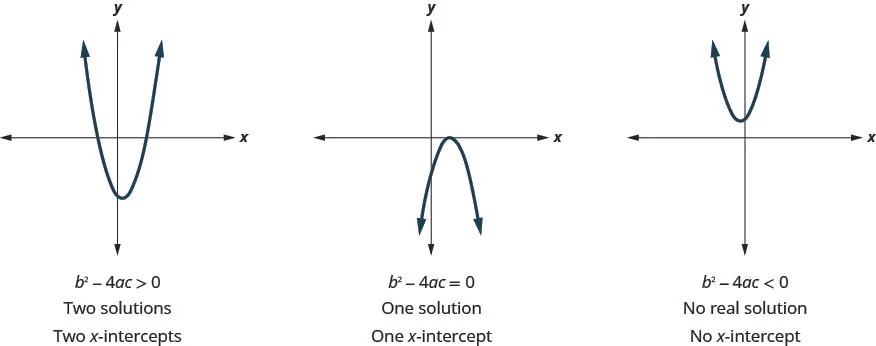Before you start solving the quadratic equation to find the values of the x-intercepts, you may want to evaluate the discriminant so you know how many solutions to expect.

### Example 10.47

Find the intercepts of the parabola $y=5x2+x+4y=5x2+x+4$.

### Try It 10.93

Find the intercepts of the parabola $y=3x2+4x+4.y=3x2+4x+4.$

### Try It 10.94

Find the intercepts of the parabola $y=x2−4x−5.y=x2−4x−5.$

### Example 10.48

Find the intercepts of the parabola $y=4x2−12x+9y=4x2−12x+9$.

### Try It 10.95

Find the intercepts of the parabola $y=−x2−12x−36.y=−x2−12x−36.$

### Try It 10.96

Find the intercepts of the parabola $y=9x2+12x+4.y=9x2+12x+4.$

### Graph Quadratic Equations in Two Variables

Now, we have all the pieces we need in order to graph a quadratic equation in two variables. We just need to put them together. In the next example, we will see how to do this.

### Example 10.49

#### How To Graph a Quadratic Equation in Two Variables

Graph $y=x2−6x+8y=x2−6x+8$.

### Try It 10.97

Graph the parabola $y=x2+2x−8.y=x2+2x−8.$

### Try It 10.98

Graph the parabola $y=x2−8x+12.y=x2−8x+12.$

### How To

#### Graph a quadratic equation in two variables.

1. Step 1. Write the quadratic equation with $yy$ on one side.
2. Step 2. Determine whether the parabola opens upward or downward.
3. Step 3. Find the axis of symmetry.
4. Step 4. Find the vertex.
5. Step 5. Find the y-intercept. Find the point symmetric to the y-intercept across the axis of symmetry.
6. Step 6. Find the x-intercepts.
7. Step 7. Graph the parabola.

We were able to find the x-intercepts in the last example by factoring. We find the x-intercepts in the next example by factoring, too.

### Example 10.50

Graph $y=−x2+6x−9y=−x2+6x−9$.

### Try It 10.99

Graph the parabola $y=−3x2+12x−12.y=−3x2+12x−12.$

### Try It 10.100

Graph the parabola $y=25x2+10x+1.y=25x2+10x+1.$

For the graph of $y=−x2+6x−9y=−x2+6x−9$, the vertex and the x-intercept were the same point. Remember how the discriminant determines the number of solutions of a quadratic equation? The discriminant of the equation $0=−x2+6x−90=−x2+6x−9$ is 0, so there is only one solution. That means there is only one x-intercept, and it is the vertex of the parabola.

How many x-intercepts would you expect to see on the graph of $y=x2+4x+5y=x2+4x+5$?

### Example 10.51

Graph $y=x2+4x+5y=x2+4x+5$.

### Try It 10.101

Graph the parabola $y=2x2−6x+5.y=2x2−6x+5.$

### Try It 10.102

Graph the parabola $y=−2x2−1.y=−2x2−1.$

Finding the y-intercept by substituting $x=0x=0$ into the equation is easy, isn’t it? But we needed to use the Quadratic Formula to find the x-intercepts in Example 10.51. We will use the Quadratic Formula again in the next example.

### Example 10.52

Graph $y=2x2−4x−3y=2x2−4x−3$.

### Try It 10.103

Graph the parabola $y=5x2+10x+3.y=5x2+10x+3.$

### Try It 10.104

Graph the parabola $y=−3x2−6x+5.y=−3x2−6x+5.$

### Solve Maximum and Minimum Applications

Knowing that the vertex of a parabola is the lowest or highest point of the parabola gives us an easy way to determine the minimum or maximum value of a quadratic equation. The y-coordinate of the vertex is the minimum y-value of a parabola that opens upward. It is the maximum y-value of a parabola that opens downward. See Figure 10.7.

Figure 10.7

### Minimum or Maximum Values of a Quadratic Equation

The y-coordinate of the vertex of the graph of a quadratic equation is the

• minimum value of the quadratic equation if the parabola opens upward.
• maximum value of the quadratic equation if the parabola opens downward.

### Example 10.53

Find the minimum value of the quadratic equation $y=x2+2x−8y=x2+2x−8$.

### Try It 10.105

Find the maximum or minimum value of the quadratic equation $y=x2−8x+12y=x2−8x+12$.

### Try It 10.106

Find the maximum or minimum value of the quadratic equation $y=−4x2+16x−11y=−4x2+16x−11$.

We have used the formula

$h=−16t2+v0t+h0h=−16t2+v0t+h0$

to calculate the height in feet, $hh$, of an object shot upwards into the air with initial velocity, $v0v0$, after $tt$ seconds.

This formula is a quadratic equation in the variable $tt$, so its graph is a parabola. By solving for the coordinates of the vertex, we can find how long it will take the object to reach its maximum height. Then, we can calculate the maximum height.

### Example 10.54

The quadratic equation $h=−16t2+v0t+h0h=−16t2+v0t+h0$ models the height of a volleyball hit straight upwards with velocity 176 feet per second from a height of 4 feet.

1. How many seconds will it take the volleyball to reach its maximum height?
2. Find the maximum height of the volleyball.

### Try It 10.107

The quadratic equation $h=−16t2+128t+32h=−16t2+128t+32$ is used to find the height of a stone thrown upward from a height of 32 feet at a rate of 128 ft/sec. How long will it take for the stone to reach its maximum height? What is the maximum height? Round answers to the nearest tenth.

### Try It 10.108

A toy rocket shot upward from the ground at a rate of 208 ft/sec has the quadratic equation of $h=−16t2+208th=−16t2+208t$. When will the rocket reach its maximum height? What will be the maximum height? Round answers to the nearest tenth.

### Media

Access these online resources for additional instruction and practice graphing quadratic equations:

### Section 10.5 Exercises

#### Practice Makes Perfect

Recognize the Graph of a Quadratic Equation in Two Variables

In the following exercises, graph:

163.

$y = x 2 + 3 y = x 2 + 3$

164.

$y = − x 2 + 1 y = − x 2 + 1$

In the following exercises, determine if the parabola opens up or down.

165.

$y = −2 x 2 − 6 x − 7 y = −2 x 2 − 6 x − 7$

166.

$y = 6 x 2 + 2 x + 3 y = 6 x 2 + 2 x + 3$

167.

$y = 4 x 2 + x − 4 y = 4 x 2 + x − 4$

168.

$y = −9 x 2 − 24 x − 16 y = −9 x 2 − 24 x − 16$

Find the Axis of Symmetry and Vertex of a Parabola

In the following exercises, find the axis of symmetry and the vertex.

169.

$y = x 2 + 8 x − 1 y = x 2 + 8 x − 1$

170.

$y = x 2 + 10 x + 25 y = x 2 + 10 x + 25$

171.

$y = − x 2 + 2 x + 5 y = − x 2 + 2 x + 5$

172.

$y = −2 x 2 − 8 x − 3 y = −2 x 2 − 8 x − 3$

Find the Intercepts of a Parabola

In the following exercises, find the x- and y-intercepts.

173.

$y = x 2 + 7 x + 6 y = x 2 + 7 x + 6$

174.

$y = x 2 + 10 x − 11 y = x 2 + 10 x − 11$

175.

$y = − x 2 + 8 x − 19 y = − x 2 + 8 x − 19$

176.

$y = x 2 + 6 x + 13 y = x 2 + 6 x + 13$

177.

$y = 4 x 2 − 20 x + 25 y = 4 x 2 − 20 x + 25$

178.

$y = − x 2 − 14 x − 49 y = − x 2 − 14 x − 49$

Graph Quadratic Equations in Two Variables

In the following exercises, graph by using intercepts, the vertex, and the axis of symmetry.

179.

$y = x 2 + 6 x + 5 y = x 2 + 6 x + 5$

180.

$y = x 2 + 4 x − 12 y = x 2 + 4 x − 12$

181.

$y = x 2 + 4 x + 3 y = x 2 + 4 x + 3$

182.

$y = x 2 − 6 x + 8 y = x 2 − 6 x + 8$

183.

$y = 9 x 2 + 12 x + 4 y = 9 x 2 + 12 x + 4$

184.

$y = − x 2 + 8 x − 16 y = − x 2 + 8 x − 16$

185.

$y = − x 2 + 2 x − 7 y = − x 2 + 2 x − 7$

186.

$y = 5 x 2 + 2 y = 5 x 2 + 2$

187.

$y = 2 x 2 − 4 x + 1 y = 2 x 2 − 4 x + 1$

188.

$y = 3 x 2 − 6 x − 1 y = 3 x 2 − 6 x − 1$

189.

$y = 2 x 2 − 4 x + 2 y = 2 x 2 − 4 x + 2$

190.

$y = −4 x 2 − 6 x − 2 y = −4 x 2 − 6 x − 2$

191.

$y = − x 2 − 4 x + 2 y = − x 2 − 4 x + 2$

192.

$y = x 2 + 6 x + 8 y = x 2 + 6 x + 8$

193.

$y = 5 x 2 − 10 x + 8 y = 5 x 2 − 10 x + 8$

194.

$y = −16 x 2 + 24 x − 9 y = −16 x 2 + 24 x − 9$

195.

$y = 3 x 2 + 18 x + 20 y = 3 x 2 + 18 x + 20$

196.

$y = −2 x 2 + 8 x − 10 y = −2 x 2 + 8 x − 10$

Solve Maximum and Minimum Applications

In the following exercises, find the maximum or minimum value.

197.

$y = 2 x 2 + x − 1 y = 2 x 2 + x − 1$

198.

$y = −4 x 2 + 12 x − 5 y = −4 x 2 + 12 x − 5$

199.

$y = x 2 − 6 x + 15 y = x 2 − 6 x + 15$

200.

$y = − x 2 + 4 x − 5 y = − x 2 + 4 x − 5$

201.

$y = −9 x 2 + 16 y = −9 x 2 + 16$

202.

$y = 4 x 2 − 49 y = 4 x 2 − 49$

In the following exercises, solve. Round answers to the nearest tenth.

203.

An arrow is shot vertically upward from a platform 45 feet high at a rate of 168 ft/sec. Use the quadratic equation $h=−16t2+168t+45h=−16t2+168t+45$ to find how long it will take the arrow to reach its maximum height, and then find the maximum height.

204.

A stone is thrown vertically upward from a platform that is 20 feet high at a rate of 160 ft/sec. Use the quadratic equation $h=−16t2+160t+20h=−16t2+160t+20$ to find how long it will take the stone to reach its maximum height, and then find the maximum height.

205.

A computer store owner estimates that by charging $xx$ dollars each for a certain computer, he can sell $40−x40−x$ computers each week. The quadratic equation $R=−x2+40xR=−x2+40x$ is used to find the revenue, $RR$, received when the selling price of a computer is $xx$. Find the selling price that will give him the maximum revenue, and then find the amount of the maximum revenue.

206.

A retailer who sells backpacks estimates that, by selling them for $xx$ dollars each, he will be able to sell $100−x100−x$ backpacks a month. The quadratic equation $R=−x2+100xR=−x2+100x$ is used to find the $RR$ received when the selling price of a backpack is $xx$. Find the selling price that will give him the maximum revenue, and then find the amount of the maximum revenue.

207.

A rancher is going to fence three sides of a corral next to a river. He needs to maximize the corral area using 240 feet of fencing. The quadratic equation $A=x(240−2x)A=x(240−2x)$ gives the area of the corral, $AA$, for the length, $xx$, of the corral along the river. Find the length of the corral along the river that will give the maximum area, and then find the maximum area of the corral.

208.

A veterinarian is enclosing a rectangular outdoor running area against his building for the dogs he cares for. He needs to maximize the area using 100 feet of fencing. The quadratic equation $A=x(100−2x)A=x(100−2x)$ gives the area, $AA$, of the dog run for the length, $xx$, of the building that will border the dog run. Find the length of the building that should border the dog run to give the maximum area, and then find the maximum area of the dog run.

#### Everyday Math

209.

In the previous set of exercises, you worked with the quadratic equation $R=−x2+40xR=−x2+40x$ that modeled the revenue received from selling computers at a price of $xx$ dollars. You found the selling price that would give the maximum revenue and calculated the maximum revenue. Now you will look at more characteristics of this model.
Graph the equation $R=−x2+40xR=−x2+40x$. Find the values of the x-intercepts.

210.

In the previous set of exercises, you worked with the quadratic equation $R=−x2+100xR=−x2+100x$ that modeled the revenue received from selling backpacks at a price of $xx$ dollars. You found the selling price that would give the maximum revenue and calculated the maximum revenue. Now you will look at more characteristics of this model.
Graph the equation $R=−x2+100xR=−x2+100x$. Find the values of the x-intercepts.

#### Writing Exercises

211.

For the revenue model in Exercise 10.205 and Exercise 10.209, explain what the x-intercepts mean to the computer store owner.

212.

For the revenue model in Exercise 10.206 and Exercise 10.210, explain what the x-intercepts mean to the backpack retailer.

#### Self Check

After completing the exercises, use this checklist to evaluate your mastery of the objectives of this section.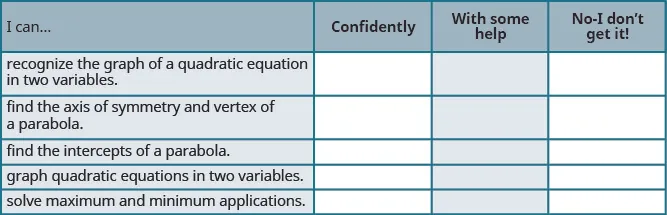What does this checklist tell you about your mastery of this section? What steps will you take to improve?

Order a print copy

As an Amazon Associate we earn from qualifying purchases.